Involutive distribution

The geometric interpretation of a completely-integrable differential system on an-dimensional differentiable manifoldof class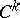,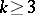. A-dimensional distribution (or a differential system of dimension) of class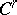,, onis a function associating to each pointa-dimensional linear subspace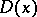of the tangent space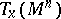such thathas a neighbourhoodwithvector fields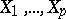on it for which the vectors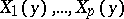form a basis of the spaceat each point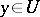. The distributionis said to be involutive if for all points,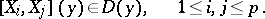This condition can also be stated in terms of differential forms. The distributionis characterized by the fact thatwhereare-forms of class, linearly independent at each point; in other words,is locally equivalent to the system of differential equations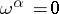. Thenis an involutive distribution if there exist-forms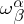onsuch thatthat is, the exterior differentials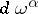belong to the ideal generated by the forms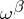.

A distributionof classon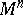is involutive if and only if (as a differential system) it is an integrable system (Frobenius' theorem).